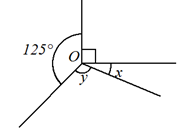### Sample Problem

The figure is not drawn to scale. ∠x = 27°. By how much is ∠x smaller than ∠y?∠x is ° smaller than ∠y.

#### Solution

The marked four angles are angles at a point, their sum is 360°.

125° + 90° + 27° + ∠y = 360°.

∠y = 360° − 125° − 90° − 27° = 118°.

∴∠y − ∠x = 118° − 27° = 91°